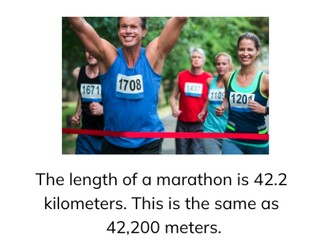Converting from m and km with decimal numbers

# Converting from m and km with decimal numbers

Students learn to convert from m and km with decimal numbers.

No account needed.8,000 schools use Gynzy92,000 teachers use Gynzy1,600,000 students use Gynzy

## General

Students learn to convert meters to kilometers and vice versa for which the number is a decimal number or becomes a decimal number.

## Standards

CCSS.Math.Content.5.MD.A.1

## Learning objective

Students will be able to convert meters (m) to kilometers (km) and vice versa with decimal numbers.

## Introduction

On the whiteboard there are several statements. If the students agree with a statement, they should stand up. If they think the statement is incorrect, they remain seated.

## Instruction

You show a map on the interactive whiteboard on which two towns are circled and the scale is provided at the bottom. Explain that you can give the distance between the two towns in different ways. Measure the distance between the towns by dragging the ruler onto the map and write down the distance in kilometers and in meters. Next you explain using a diagram of the metric system how you convert kilometers to meters. For this you take three jumps to the right. So you multiply the kilometers by one thousand. The decimal point moves three places to the right. Discuss several examples together with the class. Next the students must convert the numbers to meters. You can also convert from meters to kilometers. For this you take three jumps to the left. So you divide by one thousand. The decimal point moves three places to the left. If there is no decimal point in the number, you put it after the last digit and from there move it three places to the left. Discuss several examples together with the students. After that you have the students practice conversion on their own. Next you discuss conversion through a story problem. If you need to convert kilometers to meters, it is a multiplication problem. If you are converting meters to kilometers, it is a division problem. Have the students solve a story problem on their own.

To check whether the students can convert meters to kilometers and vice versa with decimal numbers, you can ask the following questions:
- How many meters is one kilometer?
- What do you do when you convert meters to kilometers?
- What do you do when you convert kilometers to meters?

## Quiz

The students test their understanding of converting from m and km with decimal numbers through ten exercises. In some of them they must convert meters to kilometers, and in the others they must convert kilometers to meters. Some of the exercises are in the form of a story problem.

## Closing

You discuss with the students once again that it is important to be able to convert meters to kilometers and vice versa, because that is how you can convert to a more logical unit of measurement. Next on the interactive whiteboard there are several problems, for which the students must convert the meters to kilometers and vice versa. You can have the students solve the problems and then show the answers by erasing the boxes.

## Teaching tips

When students have difficulty with converting meters and kilometers with decimal numbers, you can give them a diagram of the metric system, in which you only have meters and kilometers with an arrow for ÷1,000 and an arrow for ×1000.

## Instruction materials

You can print out a diagram of the metric system.

### The online teaching platform for interactive whiteboards and displays in schools

• Save time building lessons

• Manage the classroom more efficiently

• Increase student engagement